# Model Formulation: Goal Programming

It is important to consider model formulation before launching into the details of goal programming solutions.

Model formulation is the process of transforming a real word decision problem into an operations research model. A key to successful application of goal programming is the ability to recognize when a problem can be solved by goal programming and to formulate the corresponding model.

"Great ability develops and reveals itself increasingly with every new assignment." -Baltasar Gracian

The approach to formulate the goal programming model is similar to that of linear programming model. The mathematical model is given as follows:

Minimize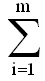wi (di + di+)

subject to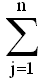aijxj + di - di+ = bi; i = 1, 2, ....., m

and xj, di, di+ ≥ 0 for all i, j

The objective function contains primarily the deviational variables (di & di+) that represent each goal or subgoal.

In LP, deviational variables are called slack or surplus variables.

The deviational variables are represented as both positive and negative deviations from each goal or subgoal. Thus, the objective function becomes the minimization of these deviations based on the relative importance or priority assigned to them.

The steps in model formulation can be summarized as follows:

##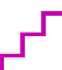Steps in Model Formulation (Algorithm): Goal Programming

1. Define Variables and Constants. The first step in model formulation is the definition of decision variables (x1,x2, ......, xn) and the right hand side constants. The right hand side constants may be either available resources or specified goal levels.
2. Formulate Constraints. The next step is to formulate a set of constraints. A constraint may be either a system constraint or a goal constraint.
3. Develop the Objective Function. Through the analysis of the decision makers goal structure, the objective function must be developed. If goals are classified in k ranks, the preemptive priority factors (symbolized by P1, P2, and so on) should be assigned to deviational variables according to their order of importance. If necessary, differential weights must be assigned to deviational variables at the same priority level.

"The reason why most major goals are not achieved is that we spend a lot of time doing second things first." -Vinay Chhabra & Manish Dewan

A lower priority goal can never be achieved at the expense of a higher priority goal.

Share and Recommend

•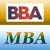MBA & BBA
•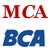BCA & MCA
•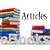Ebooks & Articles
•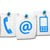Contact us